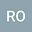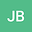Epidemiological models with quadratic equation for endemic equilibria --- a bifurcation atlas
••## Abstract

The existence and occurrence, especially by a backward bifurcation, of endemic equilibria is of utmost importance in determining the spread and persistence of a disease. In many epidemiological models, the equation for the endemic equilibria is quadratic, with the coefficients determined by the parameters of the model. Despite its apparent simplicity, such an equation can describe an amazing number of dynamical behaviours. In this paper, we shall provide a comprehensive survey of possible bifurcation patterns, deriving explicit conditions on the equation's parameters for the occurrence of each of them, and discuss illustrative examples.
02 Mar 2020Submitted to Mathematical Methods in the Applied Sciences
07 Mar 2020Assigned to Editor
07 Mar 2020Submission Checks Completed
07 Mar 2020Review(s) Completed, Editorial Evaluation Pending
08 Mar 2020Editorial Decision: Accept